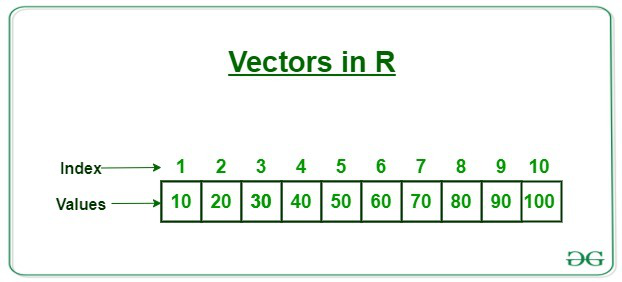Open In App

# Assigning Vectors in R Programming

Vectors are one of the most basic data structure in R. They contain data of same type. Vectors in R is equivalent to arrays in other programming languages. In R, array is a vector of one or more dimensions and every single object created is stored in the form of a vector. The members of a vector are termed as components .#### Assigning of Vectors

There are different ways of assigning vectors. In R, this task can be performed using `c()` or using “:” or using `seq()` function.

• Assigning vector using `c()`
Generally, vectors in R are assigned using `c()` function.

Example 1:

 `# R program to illustrate``# Assigning vectors`` ` `# Using c()``V = ``c``(1, 2, 4, 6, 7)`` ` `# Printing the Vector``print``(V)`` ` `# Printing the data type of the vector``print``(``typeof``(V))`

Output

``` 1 2 4 6 7
 "double"
```

Example 2:

 `# R program to illustrate``# Assigning vectors`` ` `# by default numeric values,``# double values and logical values``# are converted into characters ``V2 = ``c``(1.5, ``TRUE``, 4, ``"Geeks"``)`` ` `# Printing the Vector``print``(V2)`` ` `# Printing the data type of the vector``print``(``typeof``(V2))`

Output:

``` "1.5"   "TRUE"  "4"     "Geeks"
 "character"
```
• Assigning a vector using “:”
In R, to create a vector of consecutive values “:” operator is used.
Example 1:

 `# R program to illustrate``# Assigning vectors`` ` `# use':' to assign a vector  ``# of continuous values``V = 1:10`` ` `# Printing the vector``print``(V)`

Output

```  1  2  3  4  5  6  7  8  9 10
```

Example 2:

 `# R program to illustrate``# Assigning vectors`` ` `# use':' to assign a vector  ``# of continuous values``V = 1.5 : 9.5`` ` `# Printing the vector``print``(V)`

Output

``` 1.5 2.5 3.5 4.5 5.5 6.5 7.5 8.5 9.5
```

Example 3:

If there is a mismatch of intervals, it skips the last value.

 `# R program to illustrate``# Assigning vectors`` ` `# use':' to assign a vector  ``# of continuous values``# instead of 9.5``# here we take 9.4``V = 1.5 : 9.4`` ` `# Printing the vector``print``(V)`

Output

``` 1.5 2.5 3.5 4.5 5.5 6.5 7.5 8.5
```
• Assigning Vectors with `seq()`
In order to create vectors having step size, R provides `seq()` function.
Example 1:

 `# R program to illustrate``# Assigning vectors`` ` `# Assigning a vector using``# seq() function ``V = ``seq``(1, 3, by=0.2)`` ` `# Printing the vector``print``(V)`

Output

``` 1.0 1.2 1.4 1.6 1.8 2.0 2.2 2.4 2.6 2.8 3.0
```

Example 2:

It’s possible to specify the required length of the vector and step size is computed automatically.

 `# R program to illustrate``# Assigning vectors`` ` `# Creating a vector using seq()``# specifying the length of the vector``V = ``seq``(1, 10, length.out=5)    `` ` `# Printing the vector``print``(V)`

Output

```  1.00  3.25  5.50  7.75 10.00
```

#### Assigning Named Vectors in R

It’s also possible to create named vectors in R such that every value has a name assigned with it. R provides the `names()` function in order to create named vectors.

Example:

Suppose one wants to create a named vector with the number of players in each sport. To do so, first, he will create a numeric vector containing the number of players. Now, he can use the `names()` function to assign the name of the sports to the number of players.

 `# R program to illustrate``# Assigning named vectors`` ` `# Creating a numeric vector``# with the number of players``sports.players = ``c``(2, 4, 5, 6, 7, 9, 11)`` ` `# Assigning sports name to the numeric vector``names``(sports.players) = ``c``(``"Bridge"``, ``"Polo"``, ``"Basketball"``, ``                          ``"Volleyball"``, ``"kabaddi"``, ``                          ``"Baseball"``, ``"Cricket"``)`` ` `# Displaying the named vector``print``(sports.players)`

Output:

``` Bridge   Polo   Basketball  Volleyball  kabaddi   Baseball    Cricket

2      4            5           6        7          9         11
```

In order to get a sport with a particular number of players:

 `# Displaying the sports with 9 players``print``(``names``(sports.players[sports.players==9]))`` ` `# Displaying the sports with 1 player``print``(``names``(sports.players[sports.players==1]))`

Output:

```"Baseball"
character(0)
```

Explanation:

Baseball has nine players so it displays Baseball as output. Since here there is no sport with one player in this named vector, no output is generated and it displays the output as the character(0).

#### Access elements of a vector

In R in order to access the elements of a vector, vector indexing could be performed.

Note: Please note that indexing in R begins from 1 and not 0.

Example 1:

 `# R program ``# To access elements`` ` `# Creating a vector by seq() function``V ``=` `seq(``1``, ``40``, by``=` `4``)`` ` `# Printing the vector``print``(V)`` ` `# Printing the fifth element of the vector``print``(V[``5``])`

Output:

``` 1  5  9 13 17 21 25 29 33 37
 17
```

Example 2:

 `# R program ``# To access multiple elements`` ` `# Creating a vector by seq() function``V ``=` `seq(``1``, ``40``, by``=` `4``)`` ` `# Printing the vector``print``(V)`` ` `# Printing the fifth and seventh element of the vector``print``(V[c(``5``,``7``)])`

Output:

``` 1  5  9 13 17 21 25 29 33 37
 17 25
```